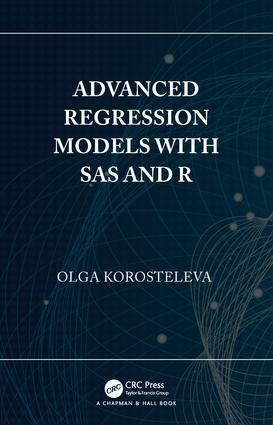# Advanced Regression Models with SAS and R

## 1st Edition

Chapman and Hall/CRC

14 pages

Hardback: 9781138049017
pub: 2018-12-10
SAVE ~\$19.99
\$99.95
\$79.96
x
eBook (VitalSource) : 9781315169828
pub: 2018-12-07
from \$49.98

FREE Standard Shipping!

### Description

Advanced Regression Models with SAS and R exposes the reader to the modern world of regression analysis. The material covered by this book consists of regression models that go beyond linear regression, including models for right-skewed, categorical and hierarchical observations. The book presents the theory as well as fully worked-out numerical examples with complete SAS and R codes for each regression. The emphasis is on model accuracy and the interpretation of results. For each regression, the fitted model is presented along with interpretation of estimated regression coefficients and prediction of response for given values of predictors.

Features:

• Presents the theoretical framework for each regression.
• Discusses data that are categorical, count, proportions, right-skewed, longitudinal and hierarchical.
• Uses examples based on real-life consulting projects.
• Provides complete SAS and R codes for each example.
• Includes several exercises for every regression.

Advanced Regression Models with SAS and R is designed as a text for an upper division undergraduate or a graduate course in regression analysis. Prior exposure to the two software packages is desired but not required.

The Author:

Olga Korosteleva is a Professor of Statistics at California State University, Long Beach. She teaches a large variety of statistical courses to undergraduate and master’s students. She has published three statistical textbooks. For a number of years, she has held the position of faculty director of the statistical consulting group. Her research interests lie mostly in applications of statistical methodology through collaboration with her clients in health sciences, nursing, kinesiology, and other fields.

1 Introduction: General and Generalized Linear Regression Models

Definition of General Linear Regression Model

Definition of Generalized Linear Regression Model

Parameter Estimation and Significance Test for Coefficients

Fitted Model

Interpretation of Estimated Regression Coefficients

Model Goodness-of-Fit Check

Predicted Response

SAS Implementation

R Implementation

Example

Exercises

2 Regression Models for Response with Right-skewed Distribution

Box-Cox Power Transformation

Model Definition

Fitted Model

Interpretation of Estimated Regression Coefficients

Predicted Response

SAS Implementation

R Implementation

Example

Gamma Regression Model

Model Definition

Fitted Model

Interpretation of Estimated Regression Coefficients

Predicted Response

SAS Implementation

R Implementation

Example

Exercises

3 Regression Models for Binary Response

Binary Logistic Regression Model

Model Definition

Fitted Model

Interpretation of Estimated Regression Coefficients

Predicted Probability

SAS Implementation

R Implementation

Example

Prohibit Model

Model Definition

Fitted Model

Interpretation of Estimated Regression Coefficients

Predicted Probability

SAS Implementation

R Implementation

Example

Complementary Log-Log Model

Model Definition and Development

Fitted Model

Interpretation of Estimated Regression Coefficients

Predicted Probability

SAS Implementation

R Implementation

Example

Exercises

4 Regression Models for Categorical Response

Cumulative Logit Model

Model Definition

Fitted Model

Interpretation of Estimated Regression Coefficients

Predicted Probabilities

SAS Implementation

R Implementation

Example

Cumulative Prohibit Model

Model Definition

Fitted Model

Interpretation of Estimated Regression Coefficients 2

Predicted Probabilities

SAS Implementation

R Implementation

Example

Cumulative Complementary Log-Log Model

Model Definition

Fitted Model

Interpretation of Estimated Regression Coefficients

Predicted Probabilities

SAS Implementation

R Implementation

Example

Generalized Logit Model for Nominal Response

Model Definition

Fitted Model

Interpretation of Estimated Regression Coefficients

Predicted Probabilities

SAS Implementation

R Implementation

Example

Exercises

5 Regression Models for Count Response

Poisson Regression Model

Model Definition

Fitted Model

Interpretation of Estimated Regression Coefficients

Predicted Response

SAS Implementation

R Implementation

Example

Zero-truncated Poisson Regression Model

Model Definition

Fitted Model

Interpretation of Estimated Regression Coefficients

Predicted Response

Implementation

R Implementation

Example

Zero-inflated Poisson Regression Model

Model Definition

Fitted Model 3

Interpretation of Estimated Regression Coefficients

Predicted Response

SAS Implementation

R Implementation

Example

Hurdle Poisson Regression Model

Model Definition

Fitted Model

Interpretation of Estimated Regression Coefficients

Predicted Response

SAS Implementation

R Implementation

Example

Exercises

6 Regression Models for Over-Dispersed Count Response

Negative Binomial Regression Model

Model Definition

Fitted Model

Interpretation of Estimated Regression Coefficients

Predicted Response

SAS Implementation

R Implementation

Example

Zero-truncated Negative Binomial Regression Model

Model Definition

Fitted Model

Interpretation of Estimated Regression Coefficients

Predicted Response

SAS Implementation

R Implementation

Example

Zero-inflated Negative Binomial Regression Model

Model Definition

Fitted Model

Interpretation of Estimated Regression Coefficients

Predicted Response

SAS Implementation

R Implementation

Example

Hurdle Negative Binomial Regression Model

Model Definition 4

Fitted Model

Interpretation of Estimated Regression Coefficients

Predicted Response

SAS Implementation

R Implementation

Example

Exercises

7 Regression Models for Proportion Response

Beta Regression Model

Model Definition

Fitted Model

Interpretation of Estimated Regression Coefficients

Predicted Response

SAS Implementation

R Implementation

Example

Zero-inflated Beta Regression Model

Model Definition

Fitted Model

Interpretation of Estimated Regression Coefficients

Predicted Response

SAS Implementation

R Implementation

Example

One-inflated Beta Regression Model

Model Definition

Fitted Model

Interpretation of Estimated Regression Coefficients

Predicted Response

SAS Implementation

R Implementation

Example

Zero-one-inflated Beta Regression Model

Model Definition

Fitted Model

Interpretation of Estimated Regression Coefficients

Predicted Response

SAS Implementation

R Implementation

Example

Exercises

8 General Linear Regression Models for Repeated Measures Data

Random Slope and Intercept Model

Model Definition

Fitted Model

Interpretation of Estimated Regression Coefficients

Model Goodness-of-Fit Check

Predicted Response

SAS Implementation

R Implementation

Example

Mixed Model with Covariance Structure for Error

Model Definition

Coefficients, and Predicted Response

Model Goodness-of-_t Check

SAS Implementation

R Implementation

Example

Generalized Estimating Equations Model

Model Definition

Fitted Model

Model Goodness-of-Fit Check

SAS Implementation

R Implementation

Example

Exercises

9 Generalized Linear Regression Model for Repeated Measures Data

Generalized Linear Mixed Model

Models Definition

Fitted Model, Interpretation, Prediction

Model Goodness-of-Fit Check

SAS Implementation

R Implementation

Example

Generalized Estimating Equations Model

Model Definition

SAS Implementation

R Implementation

Example

Exercises

10 Hierarchical Regression Model

Hierarchical Regression Model for Normal Response

Model Definition

Fitted Model

Interpretation of Estimated Regression Coefficients

Model Goodness-of-Fit Check

Predicted Response

SAS Implementation

R Implementation

Example

Hierarchical Regression Model for Other Distributions

Model Definition

Fitted Model

Interpretation of Estimated Regression Coefficients

Model Goodness-of-Fit Check

Predicted Response

SAS Implementation

R Implementation

Examples

Exercises